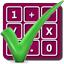#### Arithmetic of Computers

#### from Tenscope Limited

By using this site, you are accepting "session" cookies, as set out in the site's Privacy Policy
Cookies are also used to remember which page of the book you last viewed, so that when you revisit the site you automatically return to the last page you visited.

### Lesson 1

#### Page 29

34 = 3 × 3 × 3 × 3 = 81.
You are correct. The symbol 34 means “the product formed by using 3 as a factor four times.”
You can form an entire series of products by using a particular number as a factor twice, three times, four times, etc. These products are called the powers of the number. Thus:
2 × 2  = 22 = 4,   which is the 2nd power of 2,  2 × 2 × 2  = 23 = 8,   which is the 3rd power of 2,  2 × 2 × 2 × 2  = 24 = 16,   which is the 4th power of 2,
and so on.
22 can also be called “2 raised to the 2nd power,” 23 can also be called “2 raised to the 3rd power,” an so on.
The 2nd power of 3, that is, 3 raised to the 2nd power, would be the number formed by using 3 as a factor twice.
What would 5 raised to the 2nd power be?